﻿ 物理量及物理方程的时间反演变换 Time Inversion of Physical Quantity and Physical Equation

Modern Physics
Vol. 09  No. 03 ( 2019 ), Article ID: 30150 , 14 pages
10.12677/MP.2019.93018

Time Inversion of Physical Quantity and Physical Equation

—Solutions to the Problem and the Present System

Daqing Li

China Wood Luoding Cement Co. Ltd., Luoding Guangdong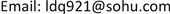Received: Apr. 17th, 2019; accepted: May 3rd, 2019; published: May 10th, 2019ABSTRACT

Based on the detailed analysis of the definition of the time inversion transformation of physical quantity, the precondition that the time inversion transformation of physical quantity leads to the reversal of the change process of its inversion quantity is found: the time inversion transformation will change the direction of the physical process under the condition that the time keeps the positive passage. Based on the definition of the new time inversion transformation, the time inversion transformation of the differential and derivative of physical quantities and the commonly used operators are analyzed in detail and important conclusions are obtained. On this basis, the time inversion transformation of several important physical equations is discussed, the time inversion transformation invariance of the equation describing the most basic physical process and the unidirectionality of the thermodynamic process independent of the time arrow are important conclusions obtained in the discussion.

Keywords:Physical Quantity, Physical Equation, The Direction of Physical Process, The Direction of Time, Time Inversion

——当前存在的问题及系统解决方案1. 引言

2. 物理量及其微分、导数的时间反演变换

2.1. 物理量的时间反演变换的定义

$Q\left({x}_{1},{x}_{2},\cdots ,{x}_{n},t\right)\to Q\left({x}_{1},{x}_{2},\cdots ,{x}_{n},{t}^{\prime }\right)=Q\left({x}_{1},{x}_{2},\cdots ,{x}_{n},-t\right)={Q}^{\prime }$ (1)

(即将Q中的时刻t用−t替换而得到一个新物理量Q′的操作)就是对Q的时间反演变换，简称T变换，该定义与物理教材中关于时间反演变换的定义一致。由定义可知，Q的T变换只是Q关于t = 0轴(或坐标原点 $O\left(0,0\right)$ )的对称变换而非Q的变化过程的逆转变换，而人们却普遍认为Q的T变换会直接无条件导致与之方向相反的物理过程(即逆过程)，并因而将其作为研究物理过程与其逆过程之间对称性的方法，这种观点很容易造成理解上的歧义，因而是有问题的并需在下面进行详细讨论。由于以下讨论将涉及物理过程的逆过程概念，为此，可将物理过程的逆过程定义为：从某一物理过程的最终状态(简称末态)开始发生的、以与该过程完全相反的方向依序经过其所有中间状态最终返回其初始状态(简称初态)的物理过程。为简化叙述，在下面的讨论中将对Q的T变换所获Q′称作Q的时间反演物理量(简称Q的反演量)，而将对Q的T变换也称作对Q所进行的、算符为 $\stackrel{^}{T}$ 的运算，即

$\stackrel{^}{T}Q\left({x}_{1},{x}_{2},\cdots ,{x}_{n},t\right)={Q}^{\prime }=Q\left({x}_{1},{x}_{2},\cdots ,{x}_{n},{t}^{\prime }\right)=Q\left({x}_{1},{x}_{2},\cdots ,{x}_{n},-t\right)$ (2)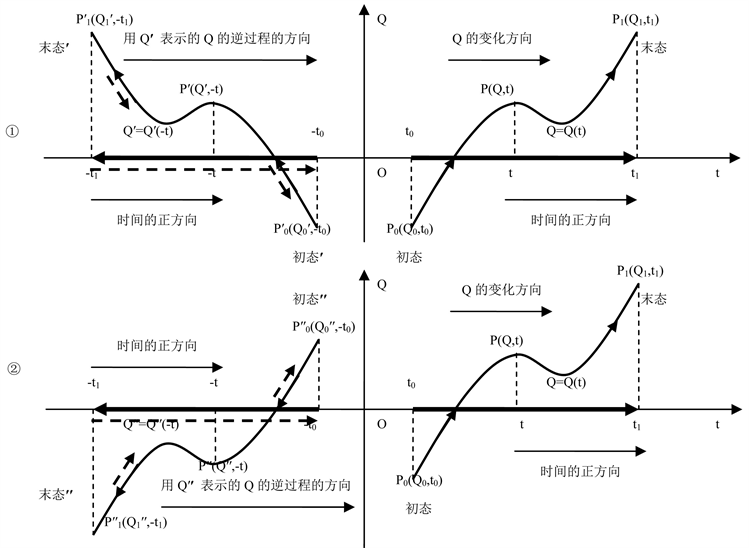Figure 1. $\stackrel{^}{T}$ operation of physical quantity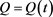2.2. 物理量的微分、导数及其常用算符的时间反演变换

$\Delta Q=Q\left({t}_{1}\right)-Q\left(t\right)=Q\left(t+\Delta t\right)-Q\left(t\right)$ (3)

$\Delta t\to {0}_{+}$，则 ${t}_{1}=t+\Delta t\to t$，则ΔQ的右极限暨Q的微分为

$\underset{\Delta t\to 0+}{\mathrm{lim}}\Delta Q=\underset{\Delta t\to 0+}{\mathrm{lim}}\left(Q\left(t+\Delta t\right)-Q\left(t\right)\right)=\text{d}Q$ (4)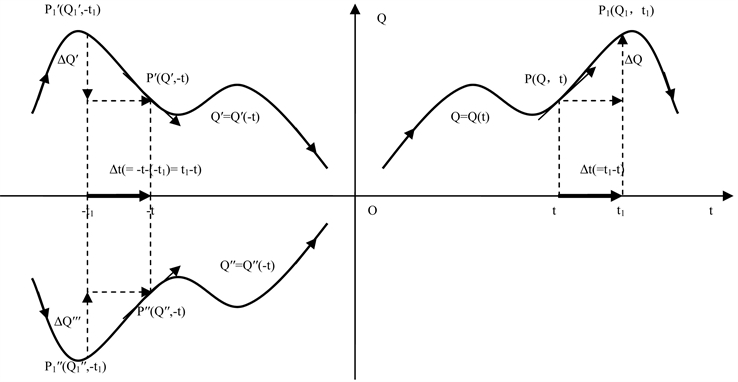Figure 2. Differentiation of $\stackrel{^}{T}$ operations of physical quantity $Q=Q\left(t\right)$

$\Delta \left(\stackrel{^}{T}Q\right)=\Delta {Q}^{\prime }=Q\left(-t\right)-Q\left(-{t}_{1}\right)=Q\left(-t\right)-Q\left(-t-\Delta t\right)$ (5)

$\Delta t\to {0}_{+}$，则 $-{t}_{1}=-t-\Delta t\to -t$，ΔQ′在时刻−t的左极限暨Q′的微分为

$\begin{array}{c}\text{d}\left(\stackrel{^}{T}Q\left(t\right)\right)=\text{d}\underset{\Delta t\to 0-}{\mathrm{lim}}\Delta {Q}^{\prime }=\underset{\Delta t\to 0-}{\mathrm{lim}}\left(Q\left(-t\right)-Q\left(-t-\Delta t\right)\right)\\ =\underset{\Delta t\to 0-}{\mathrm{lim}}\left(Q\left(t\right)-Q\left(t+\Delta t\right)\right)=-\text{d}Q\left(t\right)\end{array}$ (6)

$\text{d}\left(\stackrel{^}{T}Q\left(t\right)\right)/\text{d}t=-\text{d}Q\left(t\right)/\text{d}t$ (7)

$\text{d}\left(\stackrel{^}{T}Q\left(t\right)\right)=\text{d}Q\left(t\right)$ (8)

$\text{d}\left(\stackrel{^}{T}Q\left(t\right)\right)/\text{d}t=\text{d}Q\left(t\right)/\text{d}t$ (9)

$\frac{{\text{d}}^{2n-1}\left(\stackrel{^}{T}Q\left(t\right)\right)}{\text{d}{t}^{2n-1}}=\frac{{\text{d}}^{2n-1}Q\left({t}^{\prime }\right)}{\text{d}{\left({t}^{\prime }\right)}^{2n-1}}=\frac{-{\text{d}}^{2n-1}Q\left(t\right)}{\text{d}{t}^{2n-1}}=-\frac{{\text{d}}^{2n-1}Q\left(t\right)}{\text{d}{t}^{2n-1}}$ (10)

$\frac{{\text{d}}^{2n}\left(\stackrel{^}{T}Q\left(t\right)\right)}{\text{d}{t}^{2n}}=\frac{{\text{d}}^{2n}Q\left({t}^{\prime }\right)}{\text{d}{\left({t}^{\prime }\right)}^{2n}}=\frac{{\text{d}}^{2n}Q\left(t\right)}{\text{d}{t}^{2n}}$ (11)

$\frac{{\text{d}}^{2n-1}\left(\stackrel{^}{T}Q\left(t\right)\right)}{\text{d}{t}^{2n-1}}=\frac{{\text{d}}^{2n-1}Q\left({t}^{\prime }\right)}{\text{d}{\left({t}^{\prime }\right)}^{2n-1}}=\frac{{\text{d}}^{2n-1}Q\left(t\right)}{\text{d}{t}^{2n-1}}$ (12)

$\frac{{\text{d}}^{2n}\left(\stackrel{^}{T}Q\left(t\right)\right)}{\text{d}{t}^{2n}}=\frac{{\text{d}}^{2n}Q\left({t}^{\prime }\right)}{\text{d}{\left({t}^{\prime }\right)}^{2n}}=\frac{-{\text{d}}^{2n}Q\left(t\right)}{\text{d}{t}^{2n}}=-\frac{{\text{d}}^{2n}Q\left(t\right)}{\text{d}{t}^{2n}}$ (13)

$\frac{\partial \left(\stackrel{^}{T}Q\left({x}_{1},{x}_{2},{x}_{3},t\right)\right)}{\partial {x}_{i}}=\frac{\partial Q\left({x}_{1},{x}_{2},{x}_{3},{t}^{\prime }\right)}{\partial {x}_{i}}=±\frac{\partial Q\left({x}_{1},{x}_{2},{x}_{3},t\right)}{\partial {x}_{i}}$ (14)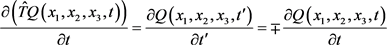(15)

$\frac{\partial \left(\stackrel{^}{T}{Q}^{2}\left({x}_{1},{x}_{2},{x}_{3},t\right)\right)}{\partial {x}_{m}\partial {x}_{n}}=\frac{\partial {Q}^{2}\left({x}_{1},{x}_{2},{x}_{3},{t}^{\prime }\right)}{\partial {x}_{m}\partial {x}_{n}}=±\frac{\partial {Q}^{2}\left({x}_{1},{x}_{2},{x}_{3},t\right)}{\partial {x}_{m}\partial {x}_{n}}$ (16)

$\frac{\partial \left(\stackrel{^}{T}{Q}^{2}\left({x}_{1},{x}_{2},{x}_{3},t\right)\right)}{\partial {t}^{2}}=\frac{\partial {Q}^{2}\left({x}_{1},{x}_{2},{x}_{3},{t}^{\prime }\right)}{\partial {{t}^{\prime }}^{2}}=±\frac{\partial {Q}^{2}\left({x}_{1},{x}_{2},{x}_{3},t\right)}{\partial {t}^{2}}$ (17)

$\frac{\partial \left(\stackrel{^}{T}{Q}^{2}\left({x}_{1},{x}_{2},{x}_{3},t\right)\right)}{\partial {x}_{i}^{2}}=\frac{\partial {Q}^{2}\left({x}_{1},{x}_{2},{x}_{3},{t}^{\prime }\right)}{\partial {x}_{i}^{2}}=±\frac{\partial {Q}^{2}\left({x}_{1},{x}_{2},{x}_{3},t\right)}{\partial {x}_{i}^{2}}$ (18)

$\nabla \cdot \left(\stackrel{^}{T}Q\right)=±\nabla \cdot Q$ (19)

$\nabla ×\left(\stackrel{^}{T}Q\right)=±\nabla ×Q$ (20)

$\stackrel{^}{T}\left(\frac{\partial }{\partial {x}_{i}}\right)=\frac{\partial }{\partial {x}_{i}}$ (21)

$\stackrel{^}{T}\left(\frac{\partial }{\partial t}\right)=\frac{{\partial }^{\prime }}{\partial {t}^{\prime }}=-\frac{\partial }{\partial t}$ (22)

$\stackrel{^}{T}\left(\frac{{\partial }^{2}}{\partial {x}_{m}\partial {x}_{n}}\right)=\frac{{\partial }^{2}}{\partial {x}_{m}\partial {x}_{n}}$ (23)

$\stackrel{^}{T}\left(\frac{{\partial }^{2}}{\partial {t}^{2}}\right)=\frac{{{\partial }^{\prime }}^{2}}{\partial {{t}^{\prime }}^{2}}=\frac{{\partial }^{2}}{\partial {t}^{2}}$ (24)

$\stackrel{^}{T}\left(\frac{{\partial }^{2}}{\partial {x}_{i}^{2}}\right)=\frac{{\partial }^{2}}{\partial {x}_{i}^{2}}$ (25)

2.3. 物理量的时间反演变换的性质

1) ${Q}_{1},{Q}_{2},\cdots ,{Q}_{n}$ 的和的 $\stackrel{^}{T}$ 运算等于 ${Q}_{1},{Q}_{2},\cdots ,{Q}_{n}$$\stackrel{^}{T}$ 运算的和，即

$\stackrel{^}{T}\left({Q}_{1}+{Q}_{2}+\cdots +{Q}_{n}\right)=\stackrel{^}{T}{Q}_{1}+\stackrel{^}{T}{Q}_{2}+\cdots +\stackrel{^}{T}{Q}_{n}$ (26)

2) ${Q}_{1},{Q}_{2},\cdots ,{Q}_{n}$ 的积的 $\stackrel{^}{T}$ 运算等于 ${Q}_{1},{Q}_{2},\cdots ,{Q}_{n}$$\stackrel{^}{T}$ 运算的积，即

$\stackrel{^}{T}\left({Q}_{1}×{Q}_{2}×\cdots ×{Q}_{n}\right)=\stackrel{^}{T}{Q}_{1}×\stackrel{^}{T}{Q}_{2}×\cdots ×\stackrel{^}{T}{Q}_{n}$ (27)

3) Q1与Q2的商的 $\stackrel{^}{T}$ 运算等于Q1与Q2$\stackrel{^}{T}$ 运算的商，即

$\stackrel{^}{T}\left({Q}_{1}/{Q}_{2}\right)=\stackrel{^}{T}{Q}_{1}/\stackrel{^}{T}{Q}_{2}$ (28)

4) Q的反演量 $\stackrel{^}{T}Q$$\stackrel{^}{T}$ 运算等于Q，即

$\stackrel{^}{T}\left(\stackrel{^}{T}Q\right)=Q$ (29)

5) Q的微分的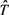运算等于Q的 $\stackrel{^}{T}$ 运算的微分，即

$\stackrel{^}{T}\left(\text{d}Q\right)=\text{d}\left(\stackrel{^}{T}Q\right)$ (30)

6) Q的导数(或偏导数)的 $\stackrel{^}{T}$ 运算等于Q的 $\stackrel{^}{T}$ 运算的导数(或偏导数)，即

$\stackrel{^}{T}\left(\text{d}Q/\text{d}t\right)=\text{d}\left(\stackrel{^}{T}Q\right)/\text{d}t$ (31)

$\stackrel{^}{T}\left(\partial Q/\partial t\right)=\partial \left(\stackrel{^}{T}Q\right)/\partial t$ (32)

$\stackrel{^}{T}\left(\partial Q/\partial {x}_{i}\right)=\partial \left(\stackrel{^}{T}Q\right)/\partial {x}_{i}$ (33)

7) Q的n阶导数(或对t的偏导数)的 $\stackrel{^}{T}$ 运算等于Q的 $\stackrel{^}{T}$ 运算的n阶导数(或对t的偏导数)，即

$\stackrel{^}{T}\left({\text{d}}^{n}Q/\text{d}{t}^{n}\right)={\text{d}}^{n}\left(\stackrel{^}{T}Q\right)/\text{d}{t}^{n}$ (34)

$\stackrel{^}{T}\left({\partial }^{n}Q/\partial {t}^{n}\right)={\partial }^{n}\left(\stackrel{^}{T}Q\right)/\partial {t}^{n}$ (35)

$\stackrel{^}{T}\left({\partial }^{n}Q/\partial {x}_{i}^{n}\right)={\partial }^{n}\left(\stackrel{^}{T}Q\right)/\partial {x}_{i}^{n}$ (36)

3. 物理方程的时间反演变换

3.1. 物理方程的时间反演变换的定义

3.2. 经典力学中主要动力学方程的时间反演变换

$\frac{\text{d}{q}_{i}}{\text{d}t}=-\frac{\partial H}{\partial {p}_{i}}$ (37)

$\frac{\text{d}{p}_{i}}{\text{d}t}=-\frac{\partial H}{\partial {q}_{i}}$ (38)

$\stackrel{^}{T}\left(\frac{\text{d}{q}_{i}}{\text{d}t}\right)=\frac{\text{d}{{q}^{\prime }}_{i}}{\text{d}{t}^{\prime }}=\frac{-\text{d}{q}_{i}}{\text{d}t}=-\frac{\text{d}{q}_{i}}{\text{d}t}$

$\stackrel{^}{T}\left(\frac{\partial H}{\partial {p}_{i}}\right)=\frac{\partial {H}^{\prime }}{\partial {{p}^{\prime }}_{i}}=\frac{-\partial H}{\partial {p}_{i}}=-\frac{\partial H}{\partial {p}_{i}}$

$\stackrel{^}{T}\left(\frac{\text{d}{p}_{i}}{\text{d}t}\right)=\frac{\text{d}{{p}^{\prime }}_{i}}{\text{d}{t}^{\prime }}=\frac{\text{d}{p}_{i}}{\text{d}t}$

$\stackrel{^}{T}\left(-\frac{\partial H}{\partial {q}_{i}}\right)=-\frac{\partial {H}^{\prime }}{\partial {{q}^{\prime }}_{i}}=-\frac{-\partial H}{-\partial {q}_{i}}=-\frac{\partial H}{\partial {q}_{i}}$

$\frac{\partial L}{\partial {q}_{i}}-\frac{\text{d}}{\text{d}t}\left(\frac{\partial L}{\partial \left(\text{d}{q}_{i}/\text{d}t\right)}\right)=0$ (39)

$\begin{array}{c}\stackrel{^}{T}\left[\frac{\partial L}{\partial {q}_{i}}-\frac{\text{d}}{\text{d}t}\left(\frac{\partial L}{\partial \left(\text{d}{q}_{i}/\text{d}t\right)}\right)\right]=\frac{\partial {L}^{\prime }}{\partial {{q}^{\prime }}_{i}}-\frac{\text{d}}{\text{d}{t}^{\prime }}\left(\frac{\partial {L}^{\prime }}{\partial \left(\text{d}{{q}^{\prime }}_{i}/\text{d ′}t\right)}\right)\\ =\frac{-\partial L}{-\partial {q}_{i}}-\frac{\text{d}}{\text{d}t}\left(\frac{-\partial L}{\partial \left(-\text{d}{q}_{i}/\text{d}t\right)}\right)\\ =\frac{\partial L}{\partial {q}_{i}}-\frac{\text{d}}{\text{d}t}\left(\frac{\partial L}{\partial \left(\text{d}{q}_{i}/\text{d}t\right)}\right)\end{array}$

3.3. 麦克斯韦电磁场方程及洛伦兹力的时间反演变换

$\nabla \cdot B=0$ (40)

$\nabla ×E=-\frac{1}{C}\frac{\partial B}{\partial t}$ (41)

$\nabla \cdot E=4\text{π}\rho$ (42)

$\nabla ×B=\frac{1}{C}\frac{\partial E}{\partial t}+\frac{4\text{π}}{C}J$ (43)

$F=eE+\frac{V}{C}e×H$ (44)

$\stackrel{^}{T}\left(\nabla \cdot B\right)=\nabla \cdot {B}^{\prime }=-\nabla \cdot B$$\stackrel{^}{T}\left(\nabla \cdot E\right)=\nabla \cdot {E}^{\prime }=\nabla \cdot E$

$\stackrel{^}{T}\left(\nabla ×E\right)=\nabla ×{E}^{\prime }=\nabla ×E$

$\stackrel{^}{T}\left(-\frac{1}{C}\frac{\partial B}{\partial t}\right)=-\frac{1}{C}\frac{\partial {B}^{\prime }}{\partial {t}^{\prime }}=-\frac{1}{C}\frac{\partial B}{\partial t}$

$\stackrel{^}{T}\left(\nabla ×B\right)=\nabla ×{B}^{\prime }=-\nabla ×B$

$\stackrel{^}{T}\left(\frac{1}{C}\frac{\partial E}{\partial t}+\frac{4\text{π}}{C}J\right)=\frac{1}{C}\frac{\partial {E}^{\prime }}{\partial {t}^{\prime }}+\frac{4\text{π}}{C}{J}^{\prime }=\frac{1}{C}\frac{\left(-\partial E\right)}{\partial t}+\frac{4\text{π}}{C}\left(-J\right)=-\frac{1}{C}\frac{\partial E}{\partial t}-\frac{4\text{π}}{C}J$

$\stackrel{^}{T}\left(eE+\left(eV/C\right)×H\right)={e}^{\prime }{E}^{\prime }+\left({e}^{\prime }{V}^{\prime }/{C}^{\prime }\right)×{H}^{\prime }=eE+\left(e\left(-V\right)/C\right)×\left(-H\right)=eE+\left(eV/C\right)×H$

3.4. 描述微观物理过程的基本方程的时间反演变换

$\hslash \frac{\partial }{\partial t}A\left(r,t\right)=\left(-\frac{{\hslash }^{2}}{2m}{\nabla }^{2}+U\left(r\right)\right)B\left(r,t\right)$ (45)

$\hslash \frac{\partial }{\partial t}B\left(r,t\right)=-\left(-\frac{{\hslash }^{2}}{2m}{\nabla }^{2}+U\left(r\right)\right)A\left(r,t\right)$ (46)

$\stackrel{^}{T}\left(A\left(r,t\right)\right)=A\left(r,{t}^{\prime }\right)=A\left(r,-t\right)=C\mathrm{cos}\left(-p\cdot r-E\left(-t\right)\right)/\hslash =A\left(r,t\right)$

$A\left(r,t\right)$$\stackrel{^}{T}$ 运算具有偶性。

$B\left(r,t\right)$$\stackrel{^}{T}$ 运算有

$\stackrel{^}{T}\left(B\left(r,t\right)\right)=B\left(r,{t}^{\prime }\right)=B\left(r,-t\right)=C\mathrm{sin}\left(-p\cdot r-E\left(-t\right)\right)/\hslash =-B\left(r,t\right)$

$B\left(r,t\right)$$\stackrel{^}{T}$ 运算具有奇性。

$\stackrel{^}{T}\left(\hslash \frac{\partial }{\partial t}A\left(r,t\right)\right)=\hslash \frac{\partial }{\partial {t}^{\prime }}A\left(r,{t}^{\prime }\right)=-\hslash \frac{\partial }{\partial t}A\left(r,t\right)$

$\stackrel{^}{T}\left(\left(-\frac{{\hslash }^{2}}{2m}{\nabla }^{2}+U\left(r\right)\right)B\left(r,t\right)\right)=\left(-\frac{{\hslash }^{2}}{2m}{\nabla }^{2}+U\left(r\right)\right)B\left(r,{t}^{\prime }\right)=-\left(-\frac{{\hslash }^{2}}{2m}{\nabla }^{2}+U\left(r\right)\right)B\left(r,t\right)$

$\stackrel{^}{T}\left(\hslash \frac{\partial }{\partial t}B\left(r,t\right)\right)=\hslash \frac{\partial }{\partial {t}^{\prime }}B\left(r,{t}^{\prime }\right)=\hslash \frac{\partial }{\partial t}B\left(r,t\right)$

$\stackrel{^}{T}\left(\left(-\frac{{\hslash }^{2}}{2m}{\nabla }^{2}+U\left(r\right)\right)A\left(r,t\right)\right)=\left(-\frac{{\hslash }^{2}}{2m}{\nabla }^{2}+U\left(r\right)\right)A\left(r,{t}^{\prime }\right)=\left(-\frac{{\hslash }^{2}}{2m}{\nabla }^{2}+U\left(r\right)\right)A\left(r,t\right)$

3.5. 描述热力学系统变化过程的物理方程的时间反演变换

$\frac{\partial T\left(X,t\right)}{\partial t}=-\lambda \frac{{\partial }^{2}T\left(X,t\right)}{\partial {X}^{2}}$ (47)

$\stackrel{^}{T}\left(\frac{\partial T\left(X,t\right)}{\partial t}\right)=\frac{\partial T\left(X,{t}^{\prime }\right)}{\partial {t}^{\prime }}=\frac{-\partial \left(T\left(X,t\right)\right)}{\partial t}=-\frac{\partial \left(T\left(X,t\right)\right)}{\partial t}$

$\stackrel{^}{T}\left(-\lambda \frac{{\partial }^{2}T\left(X,t\right)}{\partial {X}^{2}}\right)=-\lambda \frac{{\partial }^{2}T\left(X,{t}^{\prime }\right)}{\partial {X}^{2}}=-\lambda \frac{{\partial }^{2}T\left(X,t\right)}{\partial {X}^{2}}$

4. 更一般意义的时间反演变换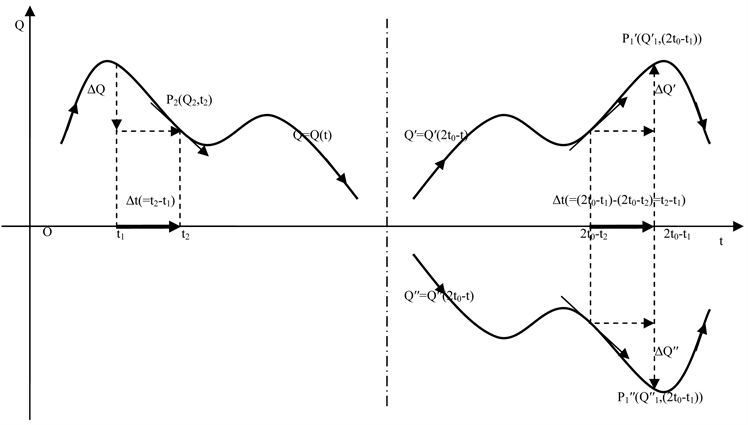Figure 3. The generalized T-transformation of the physical quantity $Q=Q\left(t\right)$ from $t={t}_{0}$ as the symmetric axis (or $P\left({t}_{0},0\right)$ to the “future”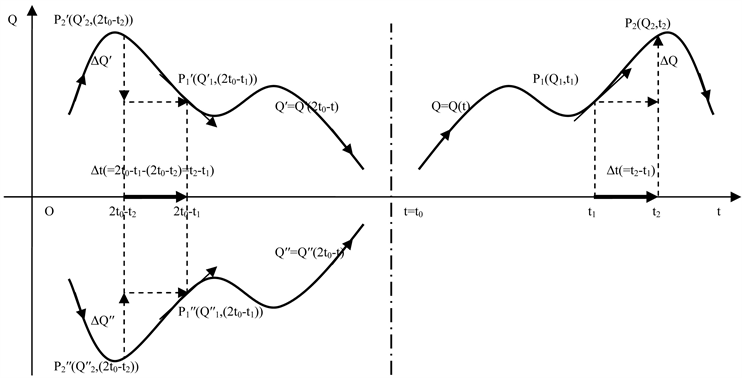Figure 4. Generalized T-transformation of physical quantity $Q=Q\left(t\right)$ from $t={t}_{0}$ as symmetric axis (or $P\left({t}_{0},0\right)$ as symmetric point) to “past”

5. 结论

1) 由于物理量的变化过程与其逆过程都是在相同的时间方向上进行的，因此，在对物理量特别是物理量的微分或导数进行 $\stackrel{^}{T}$ 运算时不能简单的将其中的时刻t用−t替换。如时刻t的 $\stackrel{^}{T}$ 运算为

$\text{d}\left(\stackrel{^}{T}t\right)=\text{d}{t}^{\prime }=\text{d}t\ne -\text{d}t$

2) 如果Q的 $\stackrel{^}{T}$ 运算具有偶性，即 $\stackrel{^}{T}Q\left(t\right)=Q\left(-t\right)=Q\left(t\right)$，则Q的 $\stackrel{^}{T}$ 运算的微分及导数的符号发生改变。特别的，如果Q的2n阶导数 ${\text{d}}^{2n}Q\left(t\right)/\text{d}{t}^{2n}$ ( $n=1,2,\cdots ,k$ )存在，则有

$\frac{{\text{d}}^{2n-1}\left(\stackrel{^}{T}Q\left(t\right)\right)}{\text{d}{t}^{2n-1}}=\frac{{\text{d}}^{2n-1}Q\left({t}^{\prime }\right)}{\text{d}{\left({t}^{\prime }\right)}^{2n-1}}=\frac{-{\text{d}}^{2n-1}Q\left(t\right)}{\text{d}{t}^{2n-1}}=-\frac{{\text{d}}^{2n-1}Q\left(t\right)}{\text{d}{t}^{2n-1}}$

$\frac{{\text{d}}^{2n}\left(\stackrel{^}{T}Q\left(t\right)\right)}{\text{d}{t}^{2n}}=\frac{{\text{d}}^{2n}Q\left({t}^{\prime }\right)}{\text{d}{\left({t}^{\prime }\right)}^{2n}}=\frac{{\text{d}}^{2n}Q\left(t\right)}{\text{d}{t}^{2n}}$

$\frac{{\text{d}}^{2n-1}\left(\stackrel{^}{T}Q\left(t\right)\right)}{\text{d}{t}^{2n-1}}=\frac{{\text{d}}^{2n-1}Q\left({t}^{\prime }\right)}{\text{d}{\left({t}^{\prime }\right)}^{2n-1}}=\frac{{\text{d}}^{2n-1}Q\left(t\right)}{\text{d}{t}^{2n-1}}$

$\frac{{\text{d}}^{2n}\left(\stackrel{^}{T}Q\left(t\right)\right)}{\text{d}{t}^{2n}}=\frac{{\text{d}}^{2n}Q\left({t}^{\prime }\right)}{\text{d}{\left({t}^{\prime }\right)}^{2n}}=\frac{-{\text{d}}^{2n}Q\left(t\right)}{\text{d}{t}^{2n}}=-\frac{{\text{d}}^{2n}Q\left(t\right)}{\text{d}{t}^{2n}}$

3) 具有 $\stackrel{^}{T}$ 运算不变性的物理方程 $F\left({x}_{1},{x}_{1},\cdots ,{x}_{n-1},{x}_{n},t\right)=0$，其函数 $F=F\left({x}_{1},{x}_{1},\cdots ,{x}_{n-1},{x}_{n},t\right)$$\stackrel{^}{T}$ 运算具有奇性或偶性，并且相应的物理过程具有可逆性。具体地说，描述最基本物理过程的方程具有 $\stackrel{^}{T}$ 运算不变性，并且相应的物理过程具有可逆性；描述热力学过程的方程不具有 $\stackrel{^}{T}$ 运算不变性，从而相应的物理过程不具有可逆性。

4) 由于物理过程与其逆过程都在正向流逝的时间方向上发生变化，因此基本物理过程的可逆性以及热力学过程的不可逆性(即单向性)均与时间的方向性无关从而并非意味时间的双向性或单向性。

5) $\stackrel{^}{T}$ 运算是研究物理过程可逆性的一种数学工具，只具有方法意义。

Time Inversion of Physical Quantity and Physical Equation[J]. 现代物理, 2019, 09(03): 162-175. https://doi.org/10.12677/MP.2019.93018

1. 1. 彼得•柯文尼, 罗杰•海菲尔德, 等. 时间之箭–解开时间最大奥秘之科学旅程[M]. 长沙: 湖南科学技术出版社, 1995: 35.

2. 2. 冯端, 冯少彤, 等. 溯源探幽熵的世界[M]. 北京: 科学出版社, 2005: 107.

3. 3. 布赖恩•格林. 宇宙的结构–空间、时间以及真实性的意义[M]. 长沙: 湖南科学技术出版社, 2012: 169.

4. 4. 布赖恩•格林. 宇宙的结构–空间、时间以及真实性的意义[M]. 长沙: 湖南科学技术出版社, 2012: 170.

5. 5. 布赖恩•格林. 宇宙的结构–空间、时间以及真实性的意义[M]. 长沙: 湖南科学技术出版社, 2012: 172.

6. 6. 刘希明. 高等量子力学[M]. 济南: 山东科学技术出版社, 2002: 229-230.

7. 7. 布赖恩•格林. 宇宙的结构–空间、时间以及真实性的意义[M]. 长沙: 湖南科学技术出版社, 2012: 159.

8. 8. 张永德. 量子力学[M]. 第4版. 北京: 科学出版社, 2017: 346.

9. 9. 尹鸿钧. 量子力学[M]. 合肥: 中国科学技术大学出版社, 1999: 455.

10. 10. 汤甦野. 熵: 一个世纪之谜的解析[M]. 第2版. 合肥: 中国科学技术大学出版社, 2008: 177.